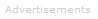# Definition of Temperature

What is Temperature?

Temperature is a quantitative measure of how hot or cold something is.Temperature is fundamentally linked to the kinetic energy of atom-scale particles. If, for example, one glass of water is measured to be hotter than another, this means its water molecules have a higher average kinetic energy than the cooler glass's molecules: the greater the average kinetic energy of the particles, the higher the temperature.

The Celsius Scale

In most scientific work, the Celsius temperature scale is used. The Celsius scale is based on the older centigrade scale, adapted slightly to take account of the absolute temperature scale, measured in kelvins, symbol K.

In approximate terms, and for the purposes of most experimental work, we can say zero degrees Celsius (0 °C) is the temperature at which water freezes and 100 °C is the temperature at which water boils at normal atmospheric pressure.

The Kelvin Scale

In thermodynamics, the kelvin scale is used. Here absolute zero is defined as zero kelvin (0 K). No lower temperature is possible. 0 K is the temperature at which all atom-scale motion ceases other than quantum fluctuations required to satisfy Heisenberg's uncertainty principle - all particle motion, as defined by pre-quantum classical physics, ceases.

At 0 K no further decrease in the average kinetic energy of particles is possible.

Comparing the Kelvin and Celsius Scales

A temperature change of 1 kelvin is identical to a change of 1 degree Celsius.

To convert a Celsius temperature to a kelvin temperature, we subtract 273.15.

• Absolute zero is: 0 K or -273.15 °C
• Water's triple point is: 273.16 K or 0.01 °C
• Water's boiling point (at 1 atm pressure) is : 373.1339 K or 99.9839 °C

The correct symbol for kelvin is K and not °K.Search the Dictionary for More Terms